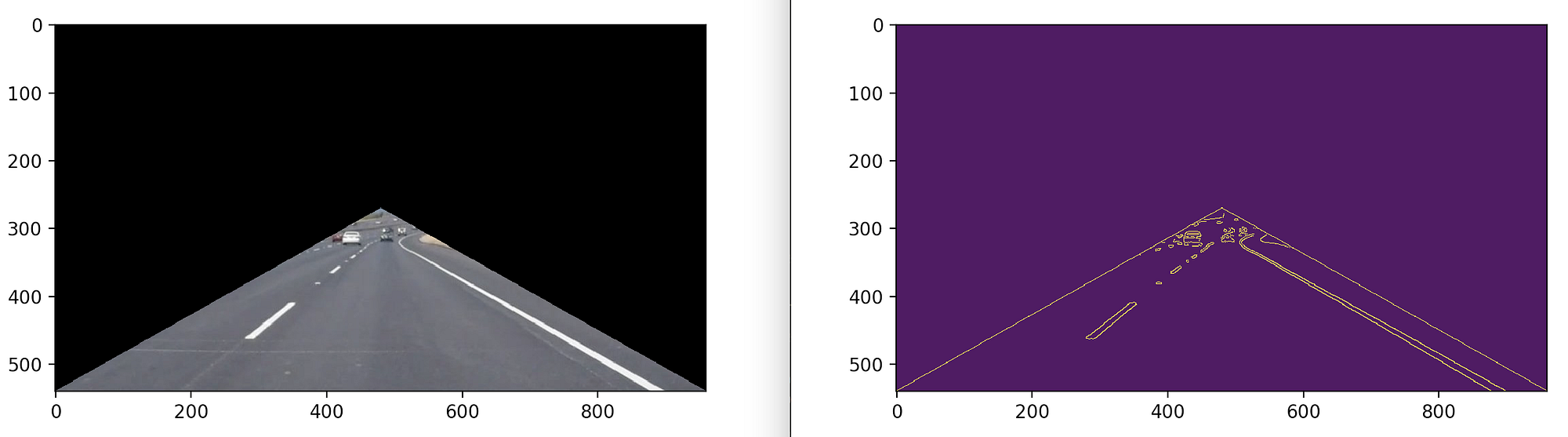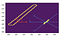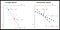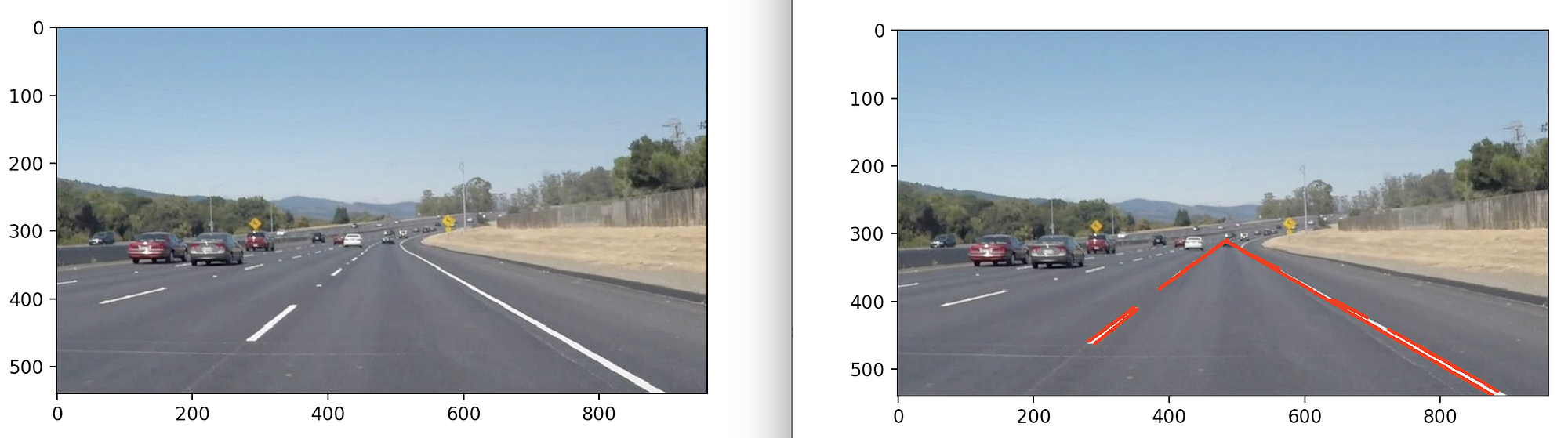# Cropping to a Region of Interest

`import matplotlib.pyplot as pltimport matplotlib.image as mpimg# reading in an imageimage = mpimg.imread('solidWhiteCurve.jpg')# printing out some stats and plotting the imageprint('This image is:', type(image), 'with dimensions:', image.shape)plt.imshow(image)plt.show()`
`\$ python load_image.pyThis image is: <class 'numpy.ndarray'> with dimensions: (540, 960, 3)`

## Defining the Region of Interest

`region_of_interest_vertices = [    (0, height),    (width / 2, height / 2),    (width, height),]`

## Cropping the Region of Interest

`import numpy as npimport cv2def region_of_interest(img, vertices):    # Define a blank matrix that matches the image height/width.    mask = np.zeros_like(img)    # Retrieve the number of color channels of the image.    channel_count = img.shape    # Create a match color with the same color channel counts.    match_mask_color = (255,) * channel_count          # Fill inside the polygon    cv2.fillPoly(mask, vertices, match_mask_color)        # Returning the image only where mask pixels match    masked_image = cv2.bitwise_and(img, mask)    return masked_image`
`import matplotlib.pyplot as pltimport matplotlib.image as mpimgregion_of_interest_vertices = [    (0, height),    (width / 2, height / 2),    (width, height),]image = mpimg.imread('solidWhiteCurve.jpg')cropped_image = region_of_interest(    image,    np.array([region_of_interest_vertices], np.int32),)plt.figure()plt.imshow(cropped_image)plt.show()`

# Detecting Edges in the Cropped Image

## Grayscale Conversion and Canny Edge Detection

`import matplotlib.pyplot as pltimport matplotlib.image as mpimgimport numpy as npimport cv2import mathdef region_of_interest(img, vertices):    mask = np.zeros_like(img)    channel_count = img.shape    match_mask_color = (255,) * channel_count    cv2.fillPoly(mask, vertices, match_mask_color)    masked_image = cv2.bitwise_and(img, mask)    return masked_imageregion_of_interest_vertices = [    (0, height),    (width / 2, height / 2),    (width, height),]image = mpimg.imread('solidWhiteCurve.jpg')cropped_image = region_of_interest(    image,    np.array([region_of_interest_vertices], np.int32),)plt.figure()plt.imshow(cropped_image)# Convert to grayscale here.gray_image = cv2.cvtColor(cropped_image, cv2.COLOR_RGB2GRAY)# Call Canny Edge Detection here.cannyed_image = cv2.Canny(gray_image, 100, 200)plt.figure()plt.imshow(cannyed_image)plt.show()`Our cropped image with edges shown as a series of many single pixels.
`def region_of_interest(img, vertices):    mask = np.zeros_like(img)    match_mask_color = 255 # <-- This line altered for grayscale.        cv2.fillPoly(mask, vertices, match_mask_color)    masked_image = cv2.bitwise_and(img, mask)    return masked_imageregion_of_interest_vertices = [    (0, height),    (width / 2, height / 2),    (width, height),]image = mpimg.imread('solidWhiteCurve.jpg')plt.figure()plt.imshow(image)plt.show()gray_image = cv2.cvtColor(image, cv2.COLOR_RGB2GRAY)cannyed_image = cv2.Canny(gray_image, 100, 200)# Moved the cropping operation to the end of the pipeline.cropped_image = region_of_interest(    cannyed_image,    np.array([region_of_interest_vertices], np.int32))plt.figure()plt.imshow(cropped_image)plt.show()`

# Generating Lines from Edge Pixels

## Mathematics of Line DetectionThe same lane marking example, post edge detection. Line candidates marked in blue.An illustration of an Image Space and it’s corresponding Hough Space (slope-intercept parameters). (Source)

## Using Hough Transforms to Detect Lines

`...image = mpimg.imread('solidWhiteCurve.jpg')gray_image = cv2.cvtColor(image, cv2.COLOR_RGB2GRAY)cannyed_image = cv2.Canny(gray_image, 200, 300)cropped_image = region_of_interest(    cannyed_image,    np.array(        [region_of_interest_vertices],        np.int32    ),)lines = cv2.HoughLinesP(    cropped_image,    rho=6,    theta=np.pi / 60,    threshold=160,    lines=np.array([]),    minLineLength=40,    maxLineGap=25)print(lines)`
`\$ python load_image.py[[[486 312 877 538]][[724 441 831 502]]...[[386 382 487 309]]]`
`[x1, y1, x2, y2]`

## Rendering Detected Hough Lines as an Overlay

`def draw_lines(img, lines, color=[255, 0, 0], thickness=3):    # If there are no lines to draw, exit.        if lines is None:            return    # Make a copy of the original image.    img = np.copy(img)    # Create a blank image that matches the original in size.    line_img = np.zeros(        (            img.shape,            img.shape,            3        ),        dtype=np.uint8,    )    # Loop over all lines and draw them on the blank image.    for line in lines:        for x1, y1, x2, y2 in line:            cv2.line(line_img, (x1, y1), (x2, y2), color, thickness)    # Merge the image with the lines onto the original.    img = cv2.addWeighted(img, 0.8, line_image, 1.0, 0.0)    # Return the modified image.    return img`
`...image = mpimg.imread('solidWhiteCurve.jpg')plt.figure()plt.imshow(image)plt.show()gray_image = cv2.cvtColor(image, cv2.COLOR_RGB2GRAY)cannyed_image = cv2.Canny(gray_image, 100, 200)cropped_image = region_of_interest(    cannyed_image,    np.array(        [region_of_interest_vertices],        np.int32    ),)lines = cv2.HoughLinesP(    cropped_image,    rho=6,    theta=np.pi / 60,    threshold=160,    lines=np.array([]),    minLineLength=40,    maxLineGap=25)line_image = draw_lines(image, lines) # <---- Add this call.plt.figure()plt.imshow(line_image)plt.show()`Output of our pipeline once we have rendered the detected lines back onto the original image.

# Creating a Single Left and Right Lane Line

## Grouping the Lines into Left and Right Groups

`...left_line_x = []left_line_y = []right_line_x = []right_line_y = []for line in lines:    for x1, y1, x2, y2 in line:        slope = (y2 - y1) / (x2 - x1) # <-- Calculating the slope.        if math.fabs(slope) < 0.5: # <-- Only consider extreme slope            continue        if slope <= 0: # <-- If the slope is negative, left group.            left_line_x.extend([x1, x2])            left_line_y.extend([y1, y2])        else: # <-- Otherwise, right group.            right_line_x.extend([x1, x2])            right_line_y.extend([y1, y2])`

## Creating a Single Linear Representation of each Line Group

`min_y = image.shape * (3 / 5) # <-- Just below the horizonmax_y = image.shape # <-- The bottom of the image`
`...poly_left = np.poly1d(np.polyfit(    left_line_y,    left_line_x,    deg=1))left_x_start = int(poly_left(max_y))left_x_end = int(poly_left(min_y))poly_right = np.poly1d(np.polyfit(    right_line_y,    right_line_x,    deg=1))right_x_start = int(poly_right(max_y))right_x_end = int(poly_right(min_y))`
`...image = mpimg.imread('solidWhiteCurve.jpg')plt.figure()plt.imshow(image)gray_image = cv2.cvtColor(image, cv2.COLOR_RGB2GRAY)cannyed_image = cv2.Canny(gray_image, 100, 200)cropped_image = region_of_interest(    cannyed_image,    np.array(        [region_of_interest_vertices],        np.int32    ),)lines = cv2.HoughLinesP(    cropped_image,    rho=6,    theta=np.pi / 60,    threshold=160,    lines=np.array([]),    minLineLength=40,    maxLineGap=25)left_line_x = []left_line_y = []right_line_x = []right_line_y = []for line in lines:    for x1, y1, x2, y2 in line:        slope = (y2 - y1) / (x2 - x1) # <-- Calculating the slope.        if math.fabs(slope) < 0.5: # <-- Only consider extreme slope            continue        if slope <= 0: # <-- If the slope is negative, left group.            left_line_x.extend([x1, x2])            left_line_y.extend([y1, y2])        else: # <-- Otherwise, right group.            right_line_x.extend([x1, x2])            right_line_y.extend([y1, y2])min_y = image.shape * (3 / 5) # <-- Just below the horizonmax_y = image.shape # <-- The bottom of the imagepoly_left = np.poly1d(np.polyfit(    left_line_y,    left_line_x,    deg=1))left_x_start = int(poly_left(max_y))left_x_end = int(poly_left(min_y))poly_right = np.poly1d(np.polyfit(    right_line_y,    right_line_x,    deg=1))right_x_start = int(poly_right(max_y))right_x_end = int(poly_right(min_y))line_image = draw_lines(    image,    [[        [left_x_start, max_y, left_x_end, min_y],        [right_x_start, max_y, right_x_end, min_y],    ]],    thickness=5,)plt.figure()plt.imshow(line_image)plt.show()`
`import matplotlib.pyplot as pltimport matplotlib.image as mpimgimport numpy as npimport cv2import mathdef region_of_interest(img, vertices):    mask = np.zeros_like(img)    match_mask_color = 255    cv2.fillPoly(mask, vertices, match_mask_color)    masked_image = cv2.bitwise_and(img, mask)    return masked_imagedef draw_lines(img, lines, color=[255, 0, 0], thickness=3):    line_img = np.zeros(        (            img.shape,            img.shape,            3        ),        dtype=np.uint8    )    img = np.copy(img)    if lines is None:        return    for line in lines:        for x1, y1, x2, y2 in line:            cv2.line(line_img, (x1, y1), (x2, y2), color, thickness)    img = cv2.addWeighted(img, 0.8, line_img, 1.0, 0.0)    return imgdef pipeline(image):    """    An image processing pipeline which will output    an image with the lane lines annotated.    """    height = image.shape    width = image.shape    region_of_interest_vertices = [        (0, height),        (width / 2, height / 2),        (width, height),    ]    gray_image = cv2.cvtColor(image, cv2.COLOR_RGB2GRAY)    cannyed_image = cv2.Canny(gray_image, 100, 200)     cropped_image = region_of_interest(        cannyed_image,        np.array(            [region_of_interest_vertices],            np.int32        ),    )     lines = cv2.HoughLinesP(        cropped_image,        rho=6,        theta=np.pi / 60,        threshold=160,        lines=np.array([]),        minLineLength=40,        maxLineGap=25    )     left_line_x = []    left_line_y = []    right_line_x = []    right_line_y = []     for line in lines:        for x1, y1, x2, y2 in line:            slope = (y2 - y1) / (x2 - x1)    if math.fabs(slope) < 0.5:        continue    if slope <= 0:        left_line_x.extend([x1, x2])        left_line_y.extend([y1, y2])    else:        right_line_x.extend([x1, x2])        right_line_y.extend([y1, y2])    min_y = int(image.shape * (3 / 5))    max_y = int(image.shape)    poly_left = np.poly1d(np.polyfit(        left_line_y,        left_line_x,        deg=1    ))     left_x_start = int(poly_left(max_y))    left_x_end = int(poly_left(min_y))     poly_right = np.poly1d(np.polyfit(        right_line_y,        right_line_x,       deg=1    ))     right_x_start = int(poly_right(max_y))    right_x_end = int(poly_right(min_y))    line_image = draw_lines(        image,        [[            [left_x_start, max_y, left_x_end, min_y],            [right_x_start, max_y, right_x_end, min_y],        ]],        thickness=5,    )    return line_image`
`from moviepy.editor import VideoFileClipfrom IPython.display import HTMLwhite_output = 'solidWhiteRight_output.mp4'clip1 = VideoFileClip("solidWhiteRight_input.mp4")white_clip = clip1.fl_image(pipeline)white_clip.write_videofile(white_output, audio=False)`